Concept wise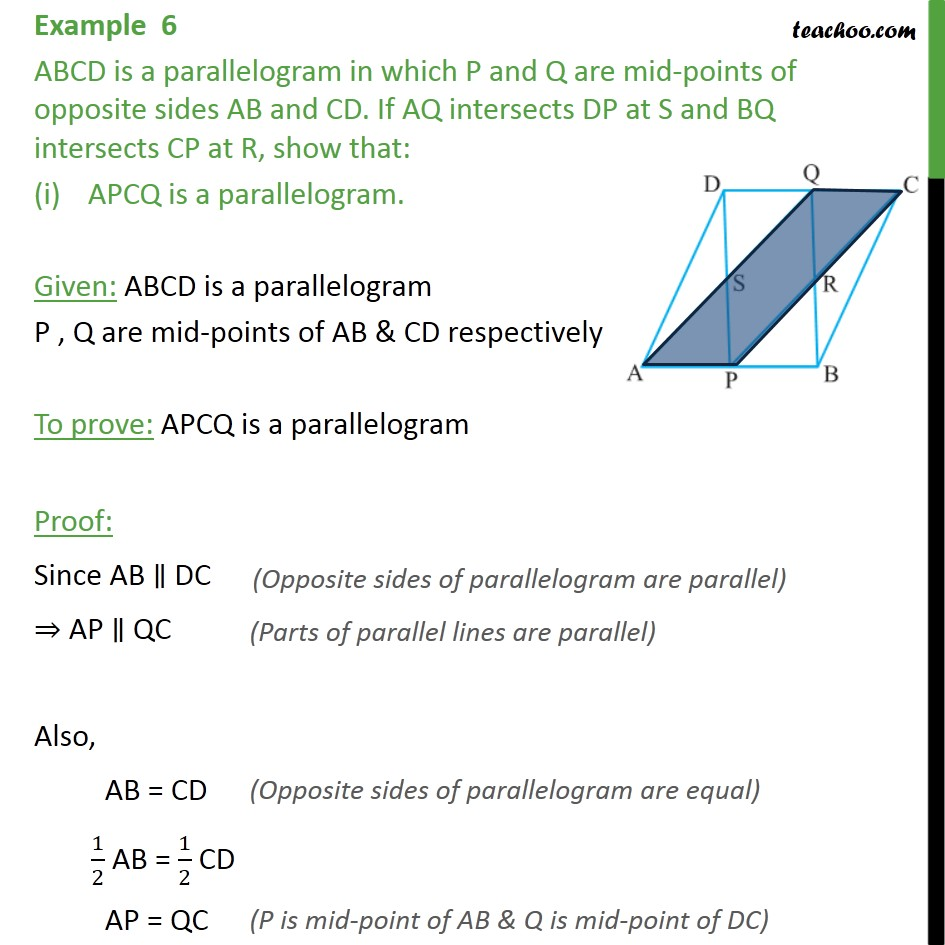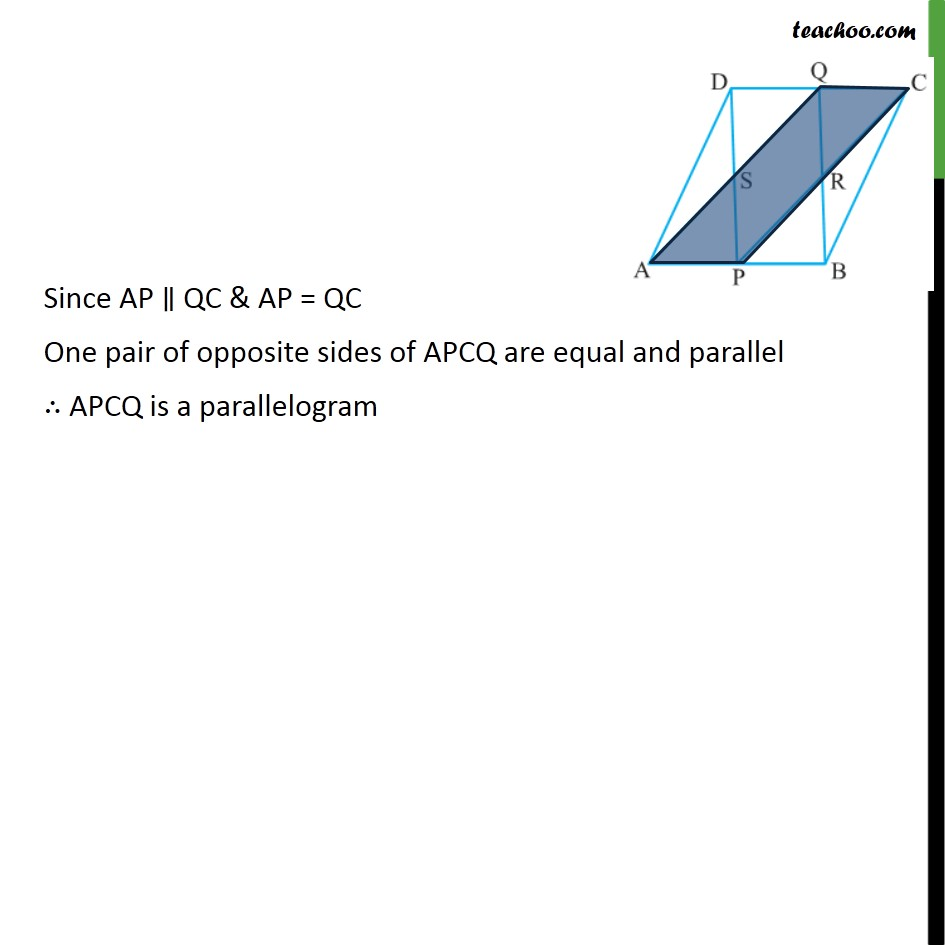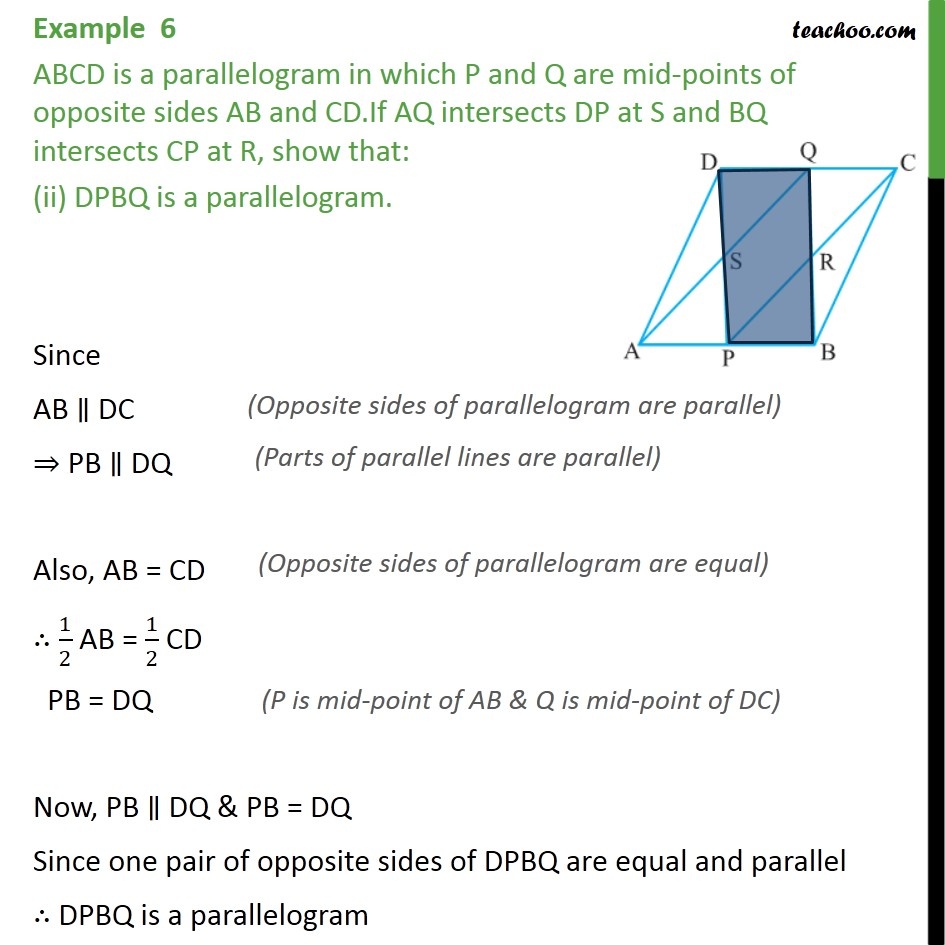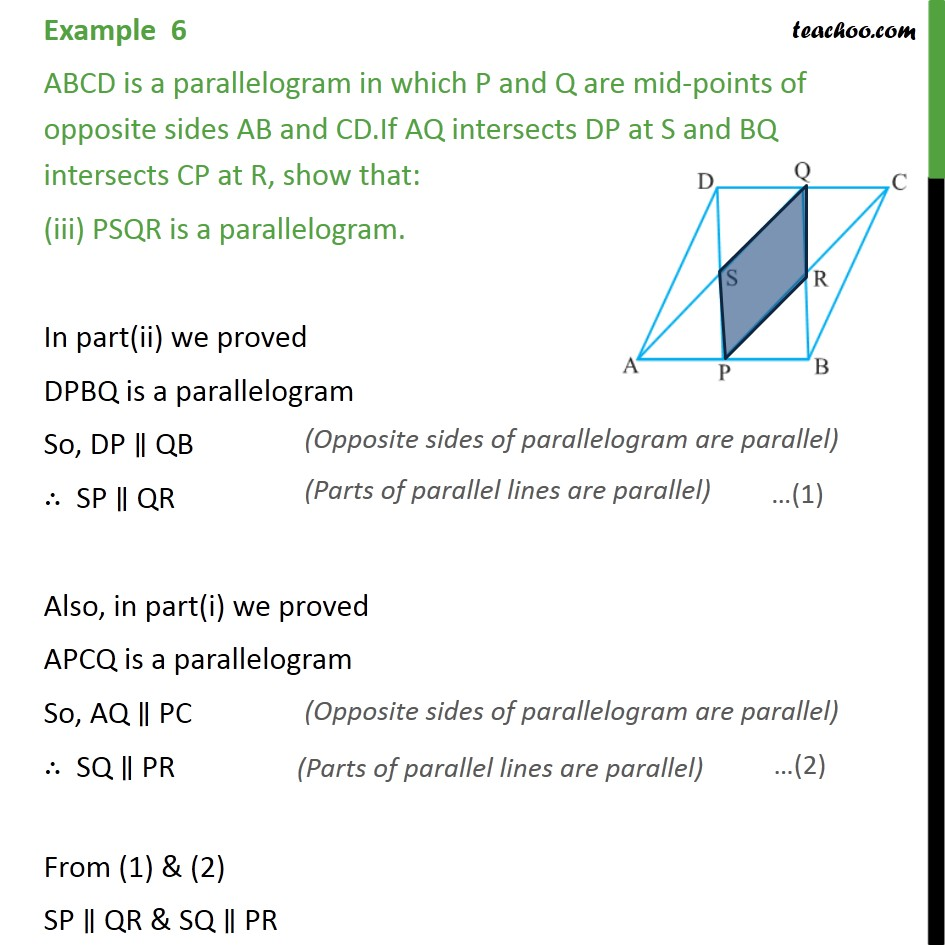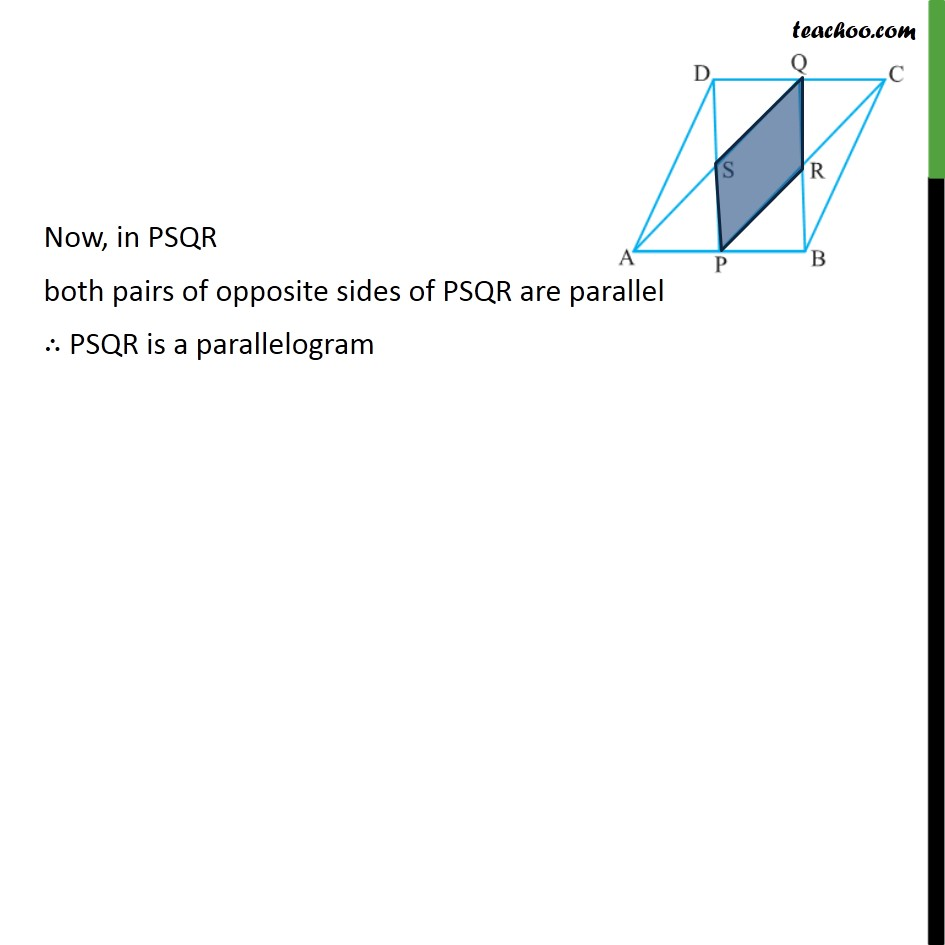Learn in your speed, with individual attention - Teachoo Maths 1-on-1 Class

### Transcript

Example 6 ABCD is a parallelogram in which P and Q are mid-points of opposite sides AB and CD. If AQ intersects DP at S and BQ intersects CP at R, show that: APCQ is a parallelogram. Given: ABCD is a parallelogram P , Q are mid-points of AB & CD respectively To prove: APCQ is a parallelogram Proof: Since AB ∥ DC ⇒ AP ∥ QC Also, AB = CD 1/2 AB = 1/2 CD AP = QC Since AP ∥ QC & AP = QC One pair of opposite sides of APCQ are equal and parallel ∴ APCQ is a parallelogram Example 6 ABCD is a parallelogram in which P and Q are mid-points of opposite sides AB and CD.If AQ intersects DP at S and BQ intersects CP at R, show that: (ii) DPBQ is a parallelogram. Since AB ∥ DC ⇒ PB ∥ DQ Also, AB = CD ∴ 1/2 AB = 1/2 CD PB = DQ Now, PB ∥ DQ & PB = DQ Since one pair of opposite sides of DPBQ are equal and parallel ∴ DPBQ is a parallelogram Example 6 ABCD is a parallelogram in which P and Q are mid-points of opposite sides AB and CD.If AQ intersects DP at S and BQ intersects CP at R, show that: (iii) PSQR is a parallelogram. In part(ii) we proved DPBQ is a parallelogram So, DP ∥ QB ∴ SP ∥ QR Also, in part(i) we proved APCQ is a parallelogram So, AQ ∥ PC ∴ SQ ∥ PR From (1) & (2) SP ∥ QR & SQ ∥ PR Now, in PSQR both pairs of opposite sides of PSQR are parallel ∴ PSQR is a parallelogram